# Two Numbered Pinecones

(Just a little obsession of mine.)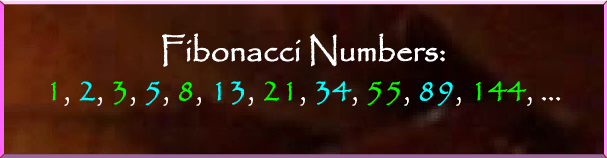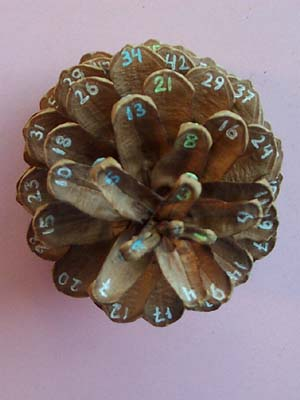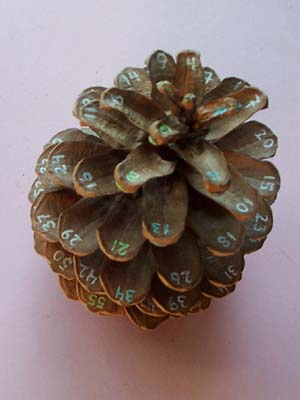I have numbered the scales of the pinecone above, found on the Ohio State University campus, in order of growth. To understand why the Fibonacci numbers (labeled in blue and green) gradually line up and how this is connected to the Fibonacci numbers of spirals at the stem end of a pinecone, see R. Knott's excellent discussion of Fibonacci Numbers and Nature, page 1 and page 2, and the references given therein. If you have access to an academic library, you can also track down my paper on the subject, “Dancing Elves and a Flower's View of Euclid's Algorithm,” in the Mathematical Intelligencer.

The essential point is that scales with numbers that differ by a Fibonacci number tend to be close together. For example, on a pinecone of this size, it is usually easy to pick out the five spirals of numbers with last digits 0 and 5, 1 and 6, 2 and 7, 3 and 8, 4 and 9, which correspond to Fibonacci differences of 5. The spirals are much clearer in three dimensions, but the tinting in the picture below may help. The physical process that accounts for these patterns and similar patterns in other plants and flowers is phyllotaxis.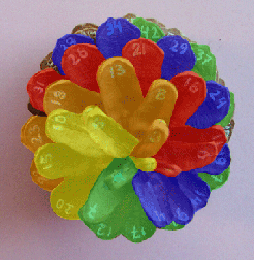I found both of the pinecones below in the woods around MSRI in Berkeley. The specimens in this batch were the first pinecones I managed to number up to 89, although I do have a number of pinecones, including this one, on which I've marked the outside of the Fibonacci scales up to 144. In the third photo, which shows the base of the open pinecone, you can see the marks on scales 89 (blue) and 144 (green). The alignment of Fibonacci scales is slightly askew near the base because of the curved spine of the pinecone, as seen in the lopsided base and in the picture of its closed cousin, fourth in the array below. Otherwise, scale 89 would be between 34 and 55.

As an illustration of the Fibonacci proximity described above, notice that if you take the numbers on any two scales that are adjacent in any direction on the pinecone, their difference is always a Fibonacci number. For instance, the scales adjacent to 50 in the first photo (clockwise from the top of the pinecone) are
 16 = 50 - 34, 37 = 50 - 13, 58 = 50 + 8, 71 = 50 + 21, 84 = 50 + 34, 63 = 50 + 13, 42 = 50 - 8, 29 = 50 - 21.
In the second photo, you can also see the proximity of 50 to 45 and to 55.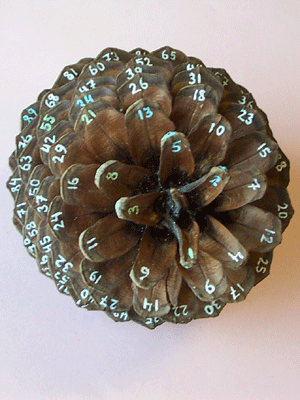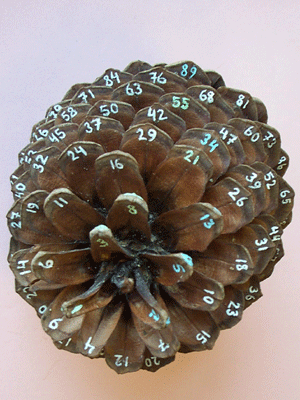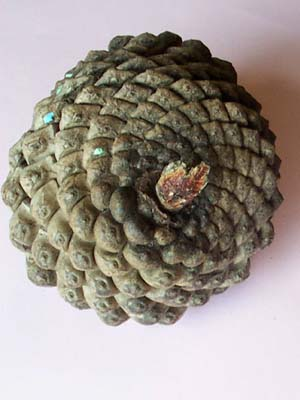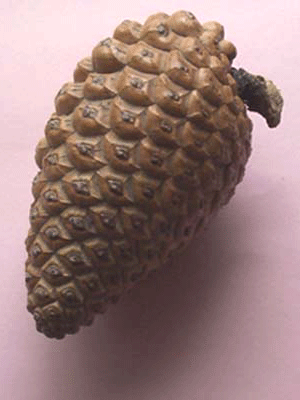In the following picture of the stem end of the large open pinecone, I have marked the family of 8 clockwise spirals in red and the family of 13 counterclockwise spirals in yellow. There is also a family of 21 clockwise spirals in white which emerges farther from the stem.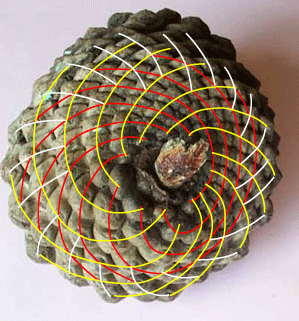The environs of St. Mary's College of Maryland are flush with straight-spined pinecones that can be numbered up to 89. Numbering them is a bit time-consuming, but if you are a mathematical hobbyist and would like to barter for numbered pinecones, I'm open to offers.

My thanks to Manuel Lladser for his assistance in taking these photographs.